1800-1023-196

+91-120-4616500

# Interstitial Sites in Close Packing

## Interstitial Sites in Close Packing

Even in the close packing of spheres, there is left some empty space between the spheres. This empty space in the crystal lattice is called site or void or hole. Voids are of following types,

(1) Trigonal void: This site is formed when three spheres lie at the vertices of an equilateral triangle. Size of the trigonal site is given by the following relation,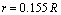r = Radius of the spherical trigonal void

R = Radius of closely packed spheres

(2) Tetrahedral void: A tetrahedral void is developed when triangular voids (made by three spheres in one layer touching each other) have contact with one sphere either in the upper layer or in the lower layer.The number of tetrahedral voids is double the number of spheres in the crystal structure.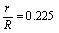where, r is the radius of the tetrahedral void or atom occupying tetrahedral void.

R is the radius of spheres forming tetrahedral void.

(3) Octahedral void: This type of void is surrounded by six closely packed spheres, i.e. it is formed by six spheres.

The number of octahedral voids is equal to the number of spheres.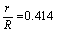(4) Cubic void: This type of void is formed between 8 closely packed spheres which occupy all the

eight corner of cube.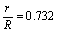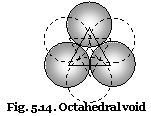The decreasing order of the size of the various voids is,

Cubic > Octahedral > Tetrahedral > Trigonal

## NEET & AIIMS Exam Sample Papers

 AIIMS SAMPLE PAPERS View More NEET SAMPLE PAPERS View More